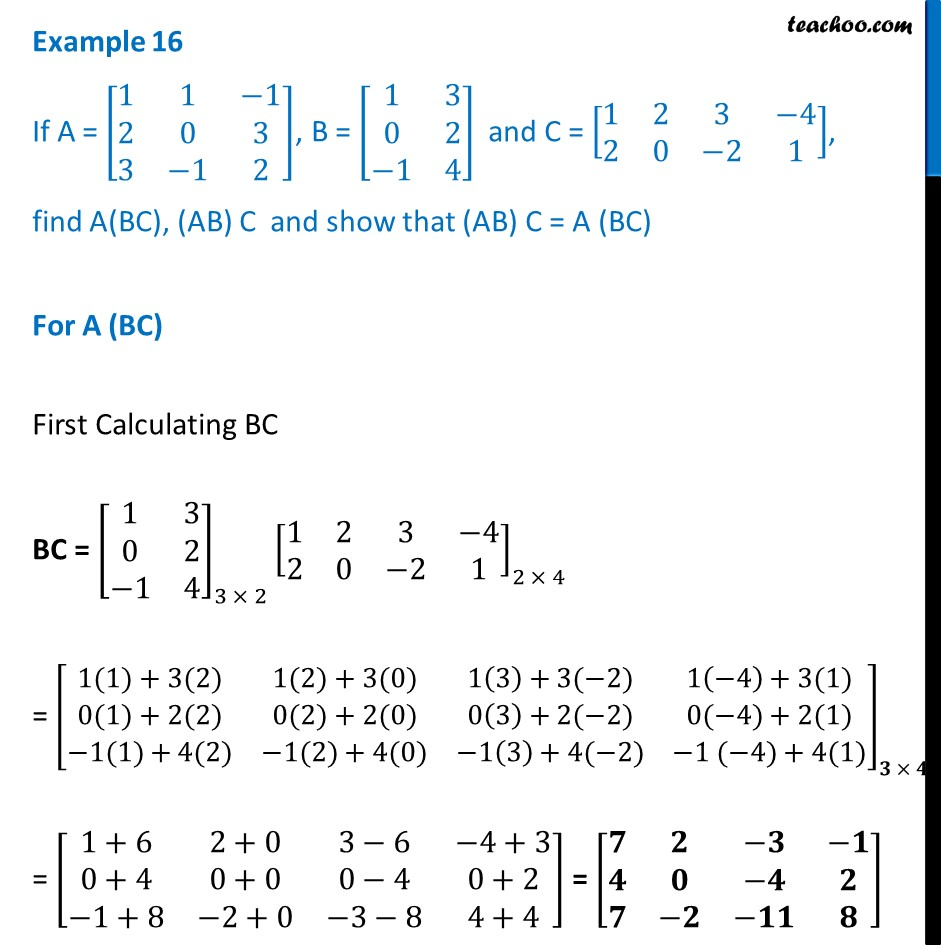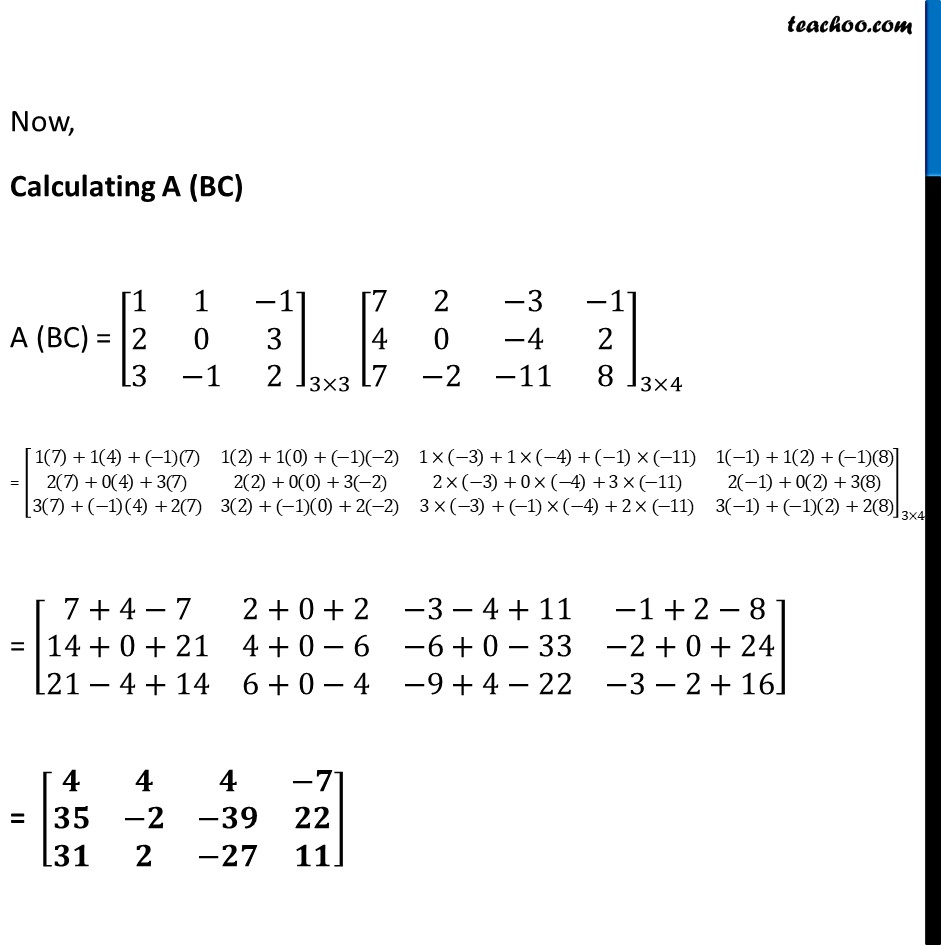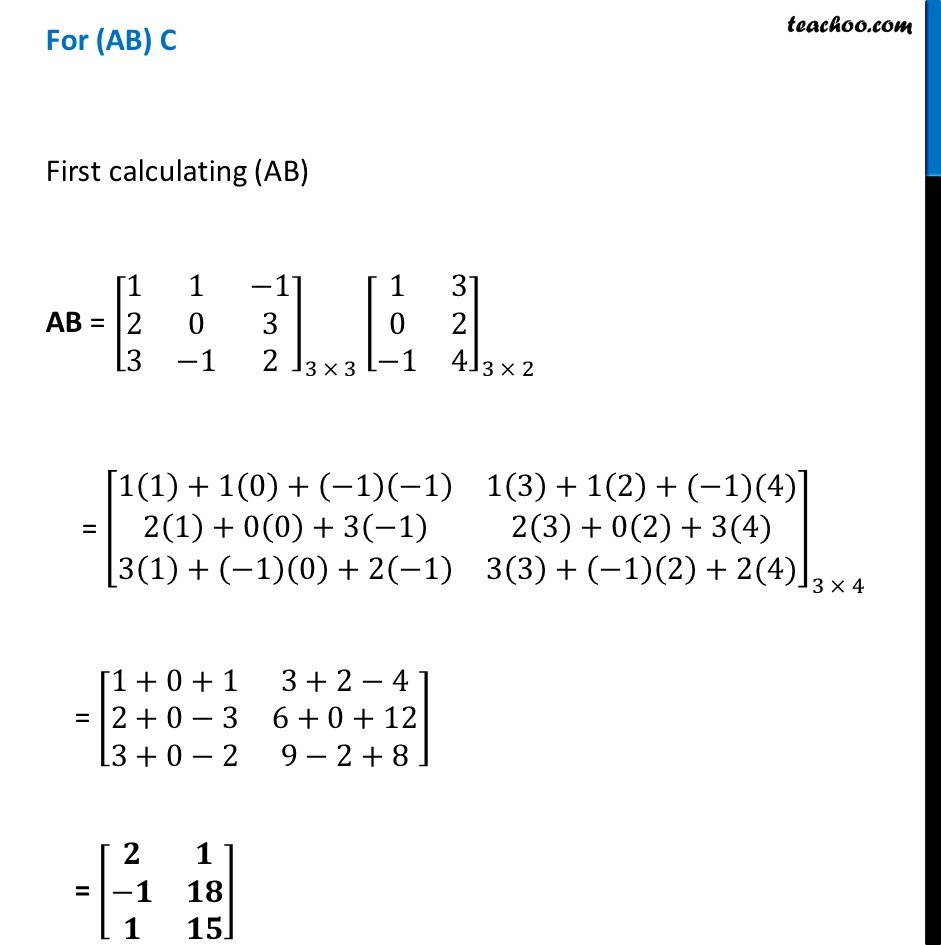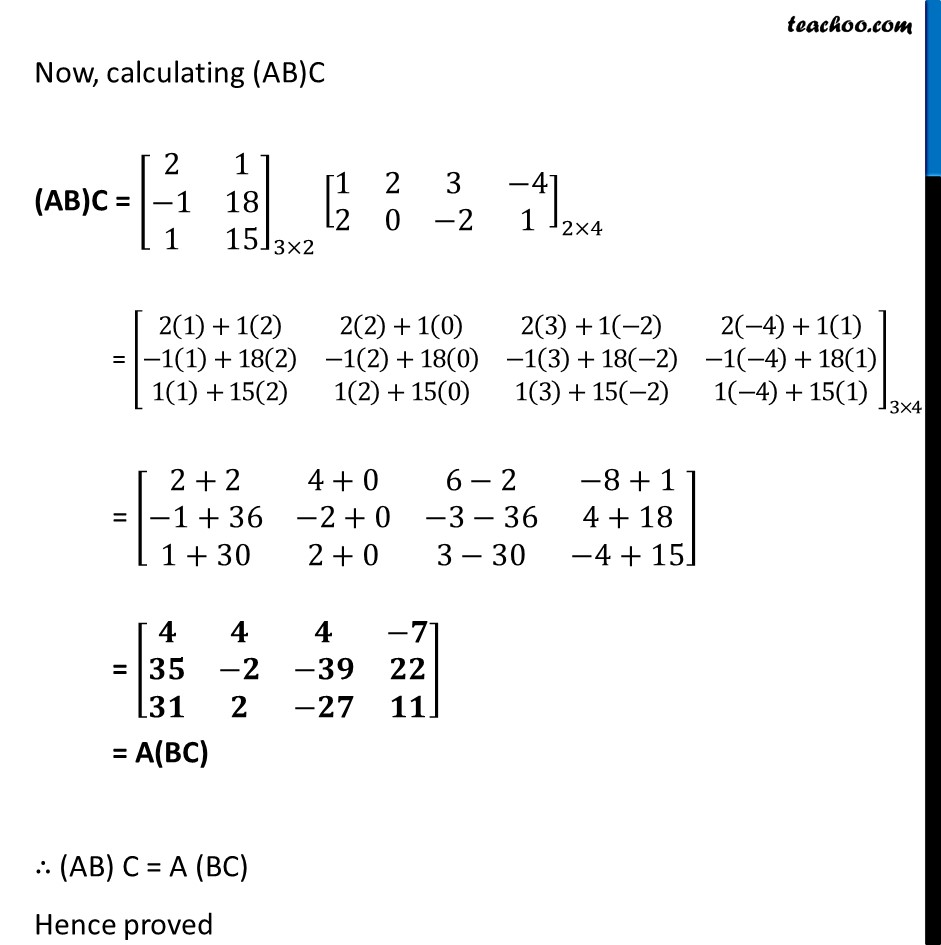Examples

Chapter 3 Class 12 Matrices
Serial order wiseLearn in your speed, with individual attention - Teachoo Maths 1-on-1 Class

### Transcript

Example 16 If A = [■8(1&1&−1@2&0&3@3&−1&2)], B = [■8(1&3@0&2@−1&4)] and C = [■8(1&2&3&−4@2&0&−2&1)], find A(BC), (AB) C and show that (AB) C = A (BC) For A (BC) First Calculating BC BC = [■8(1&3@0&2@−1&4)]_(3 × 2) [■8(1&2&3&−4@2&0&−2&1)]_(2 × 4) = [■8(1(1)+3(2)&1(2)+3(0)&1(3)+3(−2)&1(−4)+3(1)@0(1)+2(2)&0(2)+2(0)&0(3)+2(−2)&0(−4)+2(1)@−1(1)+4(2)&−1(2)+4(0)&−1(3)+4(−2)&−1 (−4)+4(1))]_(𝟑 × 𝟒) = [■8(1+6&2+0&3−6&−4+3@0+4&0+0&0−4&0+2@−1+8&−2+0&−3−8&4+4)] = [■8(𝟕&𝟐&−𝟑&−𝟏@𝟒&𝟎&−𝟒&𝟐@𝟕&−𝟐&−𝟏𝟏&𝟖)] Now, Calculating A (BC) A (BC) = [■8(1&1&−1@2&0&3@3&−1&2)]_(3×3) [■8(7&2&−3&−1@4&0&−4&2@7&−2&−11&8)]_(3×4) = [■8(1(7)+1(4)+(−1)(7)&1(2)+1(0)+(−1)(−2)&1×(−3)+1×(−4)+(−1)×(−11)&1(−1)+1(2)+(−1)(8)@2(7)+0(4)+3(7)&2(2)+0(0)+3(−2)&2×(−3)+0×(−4)+3×(−11)&2(−1)+0(2)+3(8)@3(7)+(−1)(4)+2(7)&3(2)+(−1)(0)+2(−2)&3×(−3)+(−1)×(−4)+2×(−11)&3(−1)+(−1)(2)+2(8))]_(3×4) = [■8(7+4−7&2+0+2&−3−4+11&−1+2−8@14+0+21&4+0−6&−6+0−33&−2+0+24@21−4+14&6+0−4&−9+4−22&−3−2+16)] = [■8(𝟒&𝟒&𝟒&−𝟕@𝟑𝟓&−𝟐&−𝟑𝟗&𝟐𝟐@𝟑𝟏&𝟐&−𝟐𝟕&𝟏𝟏)] For (AB) C First calculating (AB) AB = [■8(1&1&−1@2&0&3@3&−1&2)]_(3 × 3) [■8(1&3@0&2@−1&4)]_(3 × 2) = [■8(1(1)+1(0)+(−1)(−1)&1(3)+1(2)+(−1)(4)@2(1)+0(0)+3(−1)&2(3)+0(2)+3(4)@3(1)+(−1)(0)+2(−1)&3(3)+(−1)(2)+2(4))]_(3 × 4) = [■8(1+0+1&3+2−4@2+0−3&6+0+12@3+0−2&9−2+8)] = [■8(𝟐&𝟏@−𝟏&𝟏𝟖@𝟏&𝟏𝟓)] Now, calculating (AB)C (AB)C = [■8(2&1@−1&18@1&15)]_(3×2) [■8(1&2&3&−4@2&0&−2&1)]_(2×4) = [■8(2(1)+1(2)&2(2)+1(0)&2(3)+1(−2)&2(−4)+1(1)@−1(1)+18(2)&−1(2)+18(0)&−1(3)+18(−2)&−1(−4)+18(1)@1(1)+15(2)&1(2)+15(0)&1(3)+15(−2)&1(−4)+15(1) )]_(3×4) = [■8(2+2&4+0&6−2&−8+1@−1+36&−2+0&−3−36&4+18@1+30&2+0&3−30&−4+15)] = [■8(𝟒&𝟒&𝟒&−𝟕@𝟑𝟓&−𝟐&−𝟑𝟗&𝟐𝟐@𝟑𝟏&𝟐&−𝟐𝟕&𝟏𝟏)] = A(BC) ∴ (AB) C = A (BC) Hence proved